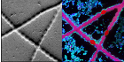#nanosims-wiki

### Sidebar

nanosims:lans_extras:pixel_by_pixel_scatter_plots

## Plotting ratios in ROIs - pixel-by-pixel in color and as 2D-histograms

2013-01-05:

I want to display an x-y scatter plot not using the ratio values derived from ion counts accumulated in ROIs but using pixel-by-pixel ratios values. I want to include only those pixels that belong to ROIs that I have drawn and classified, and I want the data-points in the scatter plot to be color-coded based on the ROI classes.

#### Possible solution

1. In the R/x and G/y fields of the main LANS GUI, specify the ID numbers of the ratios that should be used as the x and y values in the scatter plot.
2. Check Plot x-y-z graph and Pixel by pixel options in the main LANS GUI, and uncheck the ROI-averaged option.
3. Select Output → Display Ratios from the Output menu of LANS.
4. When asked, select the dat file containing the classification information of the defined ROIs.

#### Example

1. The expressions 31P/(12C+13C) and 32S/(12C+13C) are entered as the ratio expressions 3 and 4. Thus, we type 3 and 4 in the R/x and G/y fields, respectively.
2. The images of these ratios, and the corresponding pixel-by-pixel scatter plots, are shown below.

#### Few notes

Because the number of pixels in the defined ROIs is usually large, the x-y graphs may not always be very informative (e.g. they may form several indiscernible clouds). Therefore, in addition to the x-y graph, the density of the pixels in the x-y graph is also shown as a 2D histogram (see image above). The default number of bins in this 2D histogram is 50 for both x and y dimensions.